## Interpolation and least squares approximation¶

In this lecture we will consider another application of solving linear systems: approximating functions using interpolation. A function can be approximated in basis of functions $\psi_k(x)$ by finding $c_k \in \mathbb{R}$ so that $$f(x) \approx p_n(x) = \sum_{k=1}^n c_k \psi_k(x) = (\psi_1(x),\psi_2(x),\ldots,\psi_n(x))\begin{pmatrix}c_1\cr c_2\cr c_3 \cr \vdots \cr c_n \end{pmatrix}$$ We can think of this as replacing an ∞-dimensional object, the function, with a finite-dimensional object, a vector $$\mathbf{c}=\begin{pmatrix}c_1\cr c_2\cr c_3 \cr \vdots \cr c_n \end{pmatrix}$$ that can be stored on a computer.

We want to treat general smooth functions $f$, here are a plot of 4 simple example functions which we hope to be able to approximate:

In :
using PyPlot

x=-1.:0.01:1.
plot(x,exp(x))
plot(x,cos(20x.^2))   # Remember: we need .^, ./, etc. when we want to apply entrywise to a vector/matrix
plot(x,1./(25x.^2+1))
plot(x,cos(cos(x)))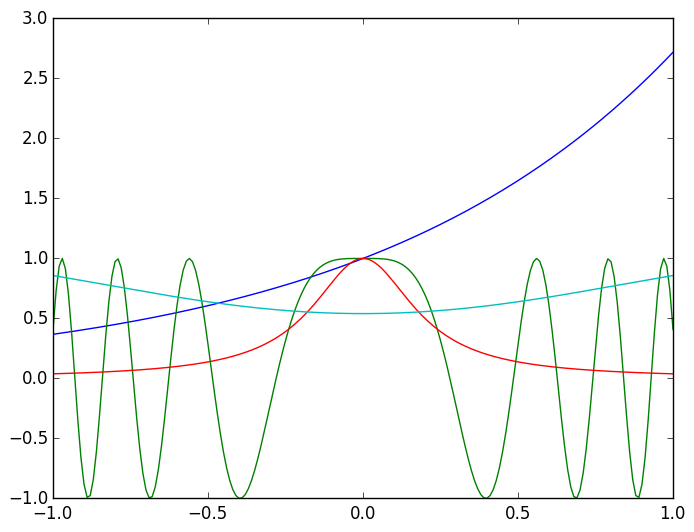Out:
1-element Array{Any,1}:
PyObject <matplotlib.lines.Line2D object at 0x319501550>

## Monomial basis¶

The classical basis are monomials:
$$(\psi_1(x),\psi_2(x),\psi_3(x),\ldots,\psi_n(x)) = (1,x,x^2,\ldots,x^{n-1})$$ One choice of $\mathbf{c}$ are the Taylor coefficients. For example, $$e^x = \sum_{k=0}^\infty {x^k \over k!} \approx \sum_{k-0}^{n-1} {x^k \over k!} = (1,x,x^2,\ldots,x^{n-1}) \begin{pmatrix}1\cr 1\cr 1/2! \cr 1/3! \cr \vdots \cr 1/(n-1)! \end{pmatrix}$$ For general functions this would turn into $$f(x) = \sum_{k=0}^\infty {f^{(k)}(0) \over k!} x^k \approx \sum_{k-0}^{n-1} {f^{(k)}(0) \over k!} x^k = (1,x,x^2,\ldots,x^{n-1}) \begin{pmatrix}f(0)\cr f'(0)\cr f''(0)/2! \cr \vdots \cr f^{(n-1)}(0)/(n-1)! \end{pmatrix}$$ For this sum to converge, we assume that Unfortunately, even if this condition is satisfied, we in general do not know the derivatives of $f$, and as we saw in Lecture 5, they are hard to compute accurately.

## Interpolation¶

In place of using derivatives, we will choose $\mathbf{c}$ so that the approximation $p_n(x)$ interpolates $f(x)$ at a sequence of points. That is, we want, at a given set of points $x_1,\ldots,x_n$, that $$p_n(x_1)=f(x_1),p_n(x_2)=f(x_2),\ldots,p_n(x_n)=f(x_n)$$ Example $$p_3(x) = 1 - {1-e^2 \over 2 e} x - {2e-e^2-1 \over 2e} x^2$$ interpolates $e^x$ at $-1,0,1$.

This is seen in the following plot, where the blue curve ($e^x$) matches the red curve ($p_3(x)$) at $-1,0,1$:

In :
using PyPlot
m=-1.:0.01:1.  # this is the plotting grid, we avoid using x to avoid confusing with the set of points x_1,…,x_n

plot(m,exp(m))
p3=1-(1-e^2)/(2e)*m-(2*e-e^2-1)/(2e)*m.^2   # evaluate p_3 at the plotting grid
plot(m,p3);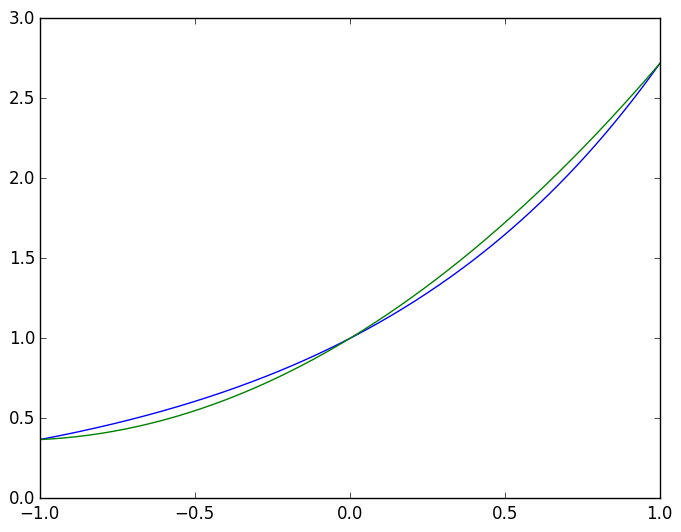We now consider calculating the interpolation for general functions. Recall that

$$p_n(x) = \sum_{k=1}^n c_k x^{k-1} = (1,x,x^2,\ldots,x^{n-1})\begin{pmatrix}c_1\cr c_2\cr c_3 \cr \vdots \cr c_n \end{pmatrix}$$

Therefore, the condition $p_n(x_1)=f(x_1),\ldots,p_n(x_n)=f(x_n)$ becomes

$$\begin{pmatrix} 1 & x_1 & x_1^2 & \cdots & x_1^{n-1} \cr 1 & x_2 & x_2^2 & \cdots & x_2^{n-1} \cr \vdots & \vdots & \ddots & \vdots \cr 1 & x_n & x_n^2 & \cdots & x_n^{n-1}\end{pmatrix} \begin{pmatrix}c_1\cr c_2\cr c_3 \cr \vdots \cr c_n \end{pmatrix} = \begin{pmatrix}f(x_1)\cr f(x_2)\cr f(x_3) \cr \vdots \cr f(x_n) \end{pmatrix}$$



Or, in other words, $$V \mathbf{c} = \mathbf{f} \qquad\hbox{for}\qquad V = \begin{pmatrix} 1 & x_1 & x_1^2 & \cdots & x_1^{n-1} \cr 1 & x_2 & x_2^2 & \cdots & x_2^{n-1} \cr \vdots & \vdots & \ddots & \vdots \cr 1 & x_n & x_n^2 & \cdots & x_n^{n-1}\end{pmatrix}\qquad\hbox{and}\qquad \mathbf{f} = \begin{pmatrix}f(x_1)\cr f(x_2)\cr f(x_3) \cr \vdots \cr f(x_n) \end{pmatrix}$$

$V$ is referred to as a Vandermonde matrix.

# Constructing a Vandermonde matrix¶

We now construct the Vandermonde matrix in Julia. We first need a grid: for now, we use $n=5$ and $$x_1 = -1, x_2 = -0.5, x_3=0., x_4=0.5, x_5=1$$ which we construct using the range syntax:

In :
x=-1.:0.5:1.
n=length(x)

Out:
5

There are multiple ways to construct $V$. The most explicit way is by looping over the entries, as follows:

In :
V=zeros(n,n)
for k=1:n
for j=1:n
V[k,j]=x[k]^(j-1)
end
end
V

Out:
5x5 Array{Float64,2}:
1.0  -1.0  1.0   -1.0    1.0
1.0  -0.5  0.25  -0.125  0.0625
1.0   0.0  0.0    0.0    0.0
1.0   0.5  0.25   0.125  0.0625
1.0   1.0  1.0    1.0    1.0   

A more succinct way is using comprehension syntax:

In :
V=[x[k]^(j-1) for k=1:n,j=1:n]    # DO NOT USE THIS!

Out:
5x5 Array{Any,2}:
1.0  -1.0  1.0   -1.0    1.0
1.0  -0.5  0.25  -0.125  0.0625
1.0   0.0  0.0    0.0    0.0
1.0   0.5  0.25   0.125  0.0625
1.0   1.0  1.0    1.0    1.0   

Warning This has not correctly inferred that the type of the matrix should be Float64: It's returned a matrix of type Any. This will break what follows, so we need to specify the type:

In :
V=Float64[x[k]^(j-1) for k=1:n,j=1:n]    # This works!

Out:
5x5 Array{Float64,2}:
1.0  -1.0  1.0   -1.0    1.0
1.0  -0.5  0.25  -0.125  0.0625
1.0   0.0  0.0    0.0    0.0
1.0   0.5  0.25   0.125  0.0625
1.0   1.0  1.0    1.0    1.0   

We can now calculate $\mathbf{c}$ by evaluating $f$ at the grid and calculiating $V^{-1} \mathbf{f}$:

In :
f=exp(x)

c=V\f

Out:
5-element Array{Float64,1}:
1.0
0.997854
0.499645
0.177347
0.0434357

We now need a function that evaluates $p_n$ at a point $x$, or vector of points $\mathbf{x}$:

In :
function p(c,x)
n=length(c)
ret=0.0
for k=1:n
ret=ret+c[k]*x.^(k-1)   # use .^ to allow x to be a vector
end
ret
end

Out:
p (generic function with 1 method)

We can check the accuracy:

In :
p(c,0.1)-exp(0.1)

Out:
-0.00020740269041175097

If we plot the difference, we see that they are indistinguishable to the eye:

In :
m=-1.:0.001:1.  # need fine plotting grid to see difference between f and p
plot(m,exp(m))
plot(m,p(c,m));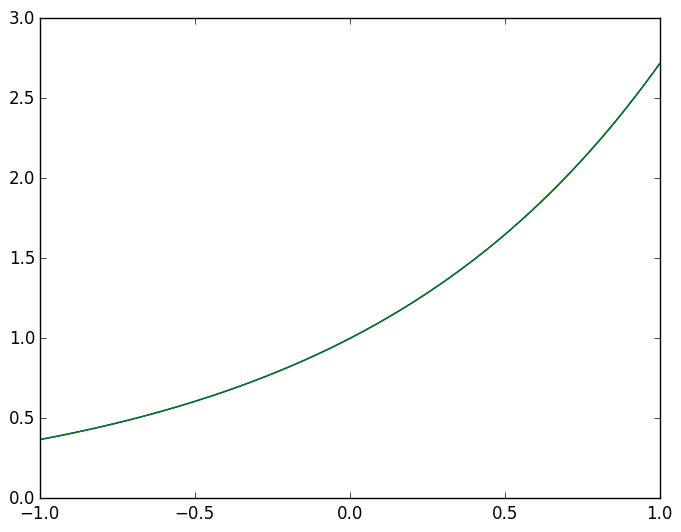Let's try increasing the number of points:

In :
x=-1.:0.1:1.
n=length(x)
V=Float64[x[k]^(j-1) for k=1:n,j=1:n]
f=exp(x)
c=V\f

Out:
21-element Array{Float64,1}:
1.0
1.0
0.5
0.166667
0.0416667
0.00833333
0.00138889
0.000198413
2.48013e-5
2.75551e-6
2.77302e-7
2.57703e-8
-3.70528e-9
-1.20658e-9
1.16819e-8
1.52576e-9
-1.37884e-8
-9.18341e-10
8.75891e-9
2.29939e-10
-2.29939e-9 

We are correctly calculating the Taylor coefficients, at least for the first few!

In :
Float64[1/factorial(k) for k=0:n-1]

Out:
21-element Array{Float64,1}:
1.0
1.0
0.5
0.166667
0.0416667
0.00833333
0.00138889
0.000198413
2.48016e-5
2.75573e-6
2.75573e-7
2.50521e-8
2.08768e-9
1.6059e-10
1.14707e-11
7.64716e-13
4.77948e-14
2.81146e-15
1.56192e-16
8.22064e-18
4.11032e-19

Let's try another example. We'll use linspace to create a range with exactly $n$ points.

In :
m=-1.:0.001:1.  # plotting grid

plot(m,1./(25m.^2+1))  # the true function

for n=5:5:20  # plot the interpolant for difference choices of n
x=linspace(-1.,1.,n)
V=Float64[x[k]^(j-1) for k=1:n,j=1:n]
f=1./(25x.^2+1)
c=V\f
plot(m,p(c,m))
end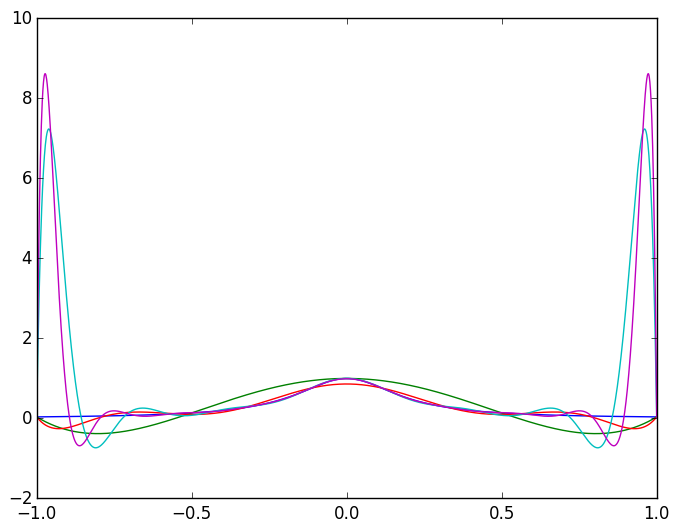As we increase $n$, the interpolant gets worse and worse near ±1!

We see a similar effect for $e^x$ if we over resolve the function:

In :
x=linspace(-1.,1.,200)

n=length(x)
V=Float64[x[k]^(j-1) for k=1:n,j=1:n]  # we need to specify the type

f=exp(x)
c=inv(V)*f

m=-1.:0.001:1.
plot(m,exp(m))
plot(m,p(c,m));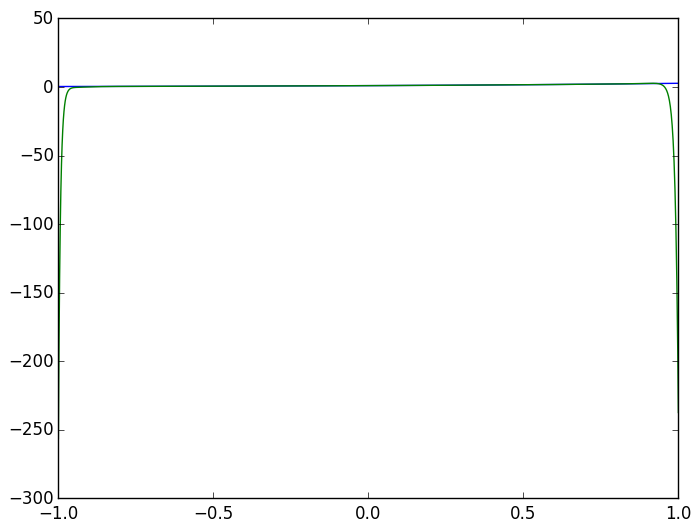Next lecture we will look at ways to resolve this issue.